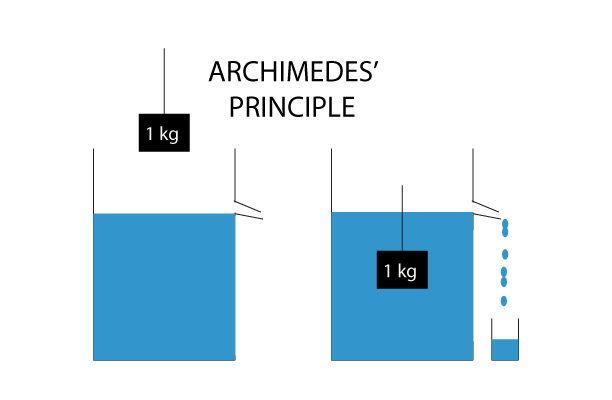# Archimedes’ Principle

`When a stone is immersed in a bucket completely filled with water, some amount of water spill out of the bucket. The weight of water displaced (spilled) from the bucket can be explained by Archimedes principle.`## Statement of Archimedes’ Principle

Archimedes’ principle states that

When an object is fully or partially immersed in a fluid, it experience an upthrust which is equal to the weight of liquid displaced by that object.

Let us consider, a stone (weight 15N) is immersed in water. Suppose, 5N water is displayed by the stone. Then, the force it exerts on the string is:

```Force on String = Weight of stone - weight of water displayed
= 15N - 5N
= 10N```

This upward force reduces the apparent weight of the stone. This is why, it is easier to lift object inside water.

### Archimedes’ Formula

For a body which is completely submerged in water the formula using Archimedes’ principle is given by:

`apparent weight of object = weight of object - weight of displayed fluid`

Another formula for a body immersed in water is:
\$\$frac{density, of, object}{density, of, fluid} = frac{weight, of, object}{weight, of, displaced, object}\$\$

Now, the above formulae can be reformulated as
\$\$frac{density , of , object}{density, of, fluid}= frac{weight, of, object}{weight, of , object-apparent , weight, of, object}\$\$

For a body floating on the surface of a liquid the formula isf

\$\$mathit{weight, of , object = weight, of , displaced , fluid}\$\$

## Principle of flotation

We already know that Archimedes Principle shows us the upward force exerted on the body which is equal to the weight of the fluid displaced. But this concept can also be used to know why some objects float on the surface of fluids.

Law of flotation states that,

For a floating object, the weight of the object equals the weight of the liquid displaced by the object.

As we have already written on the formula section of this blog we know, a body floating on the surface of a fluid, the weight of the object is equal to the weight of the displaced fluid. In order for an object to be afloat on the surface of a fluid, its density must be less than that of the fluid.

Let us take a solid iron block whose density is 7874 kg/m3 which is almost 8 times more than that of water whose density is 1000kg/m3. When this solid iron is immersed in water then only 18 of its weight of water is displaced and the iron sinks in the water. If it is reshaped and built in such a way that the displaced water is equal to the weight of the water block then in such case the iron block will float on the surface of water. This same principle is used in making of the ships.

This is true for objects floating in air as well. A balloon filled with helium floats in air as the density of helium is less than that of air.

Note: The displaced volume is directly proportional to the volume of object and not to mass of the object.

## Applications of Archimedes Principle

1. Hot air balloon
The upward force acting on a hot air ballon is greater than that the surrounding air as the density of hot air is less than that of cold air. Due to this reason it floats in the air. In order to ascend higher one simply needs to increase the heat inside the hot air balloon which results in higher buoyant force acting on the balloon. Similarly in order to descend one just simply needs to decrease the heat inside the hot air balloon.
2. Hydrometer
A hydrometer is an instrument which is used to measure the relative density of liquids. In a hydrometer the liquid rises vertically by which we can know the density of liquid in its reading. The higher the density of the liquid the higher rise in the reading level of the hydrometer.
3. Ship
Although ships are made up of metals whose density is remarkably higher than that of water it is made in such a way that its weight is equal to the weight of the water displaced by it.